# Analytics Calculations: To First Subcategory

Moderator, Lightspeed Staff Posts: 87 moderator
edited July 2018
Sometimes Analytics may be used to prepare data for further analysis in programs such as Excel or Numbers. This section of discussions touches on ways to maximize data-preparation within Analytics to facilitate the next steps

Analytics will let you add a dimension of "Top Level" category, or will let you add a dimension of the full category, including its complete subcategory. Currently there is not a way to have Analytics report on only the first subcategory, but we can add a calculation to change the full category into just the top-level and secondary subcategory.

Let's start our report by looking at recent sales: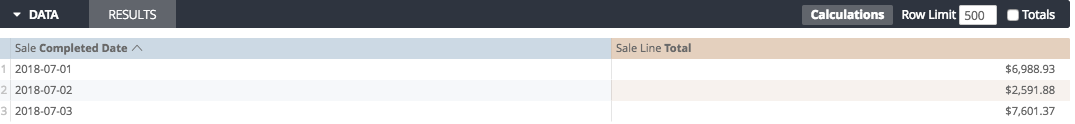And let's change the dimensions on the report from "Completed Date" to "Category"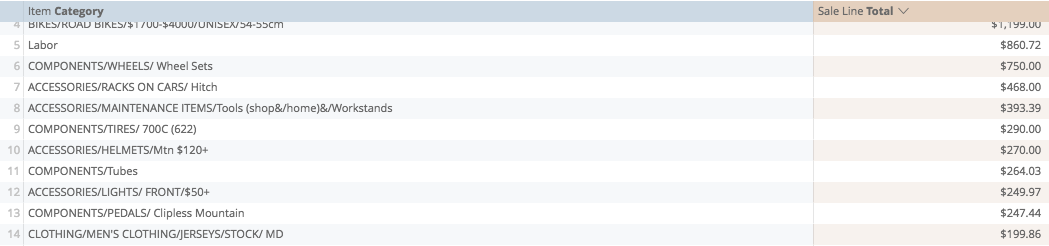Some of these categories are one layer deep, some are two layers deep, some even go further.

What we want to do, from our cell-based functions, is use the "Substring" function to slice a category at a very precise location, before its second forward slash. The trick now is to determine with calculations if there is a second slash, and if so, where?

This is the best way I have found yet*:
• What is the length of the first category?
• If there is a forward slash following this, what is its length?
• What is the length of the first sub-category?

## Step OneWhat is the length of the first category?

Let's start by using the "Position" function. Position requires two arguments: which column we are looking at, and what content we are looking for.

In this case, we're looking at the Category...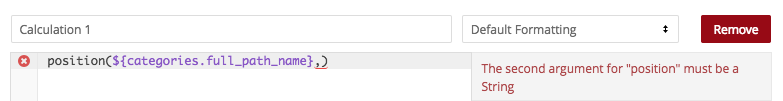...for the forward slash, as this is how Retail distinguishes hierarchy between category levels...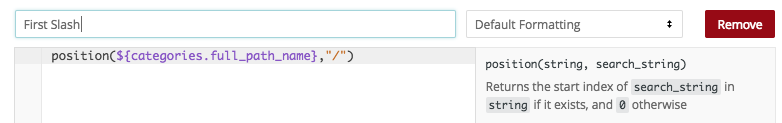So when we save...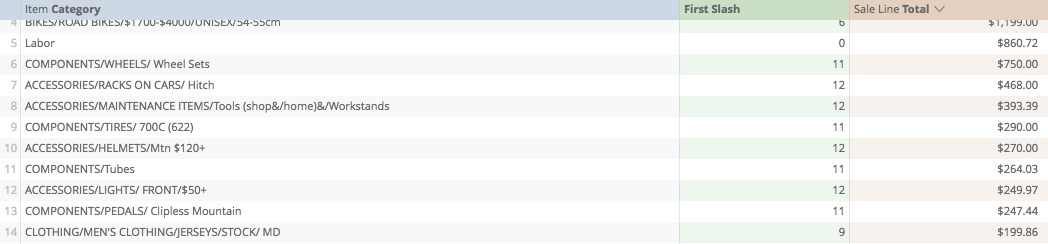We get a number showing us where the first slash occurs.

Some of the categories here no forward slash at all, such as "Labor" in the example above. In these cases, we determine the length of the first word by simply using the "Length" function.

For the categories that do have a forward slash, the calculation above is not showing us how long the word is, but the length of the first word and the forward slash.

"Components" for example, is 10 letters long, the forward slash is the 11th character in the string.

So we can build an if calculation to determine what to do next.

If the first slash is zero, length, if not, the position of the first slash minus 1.

Let's start simply with brackets...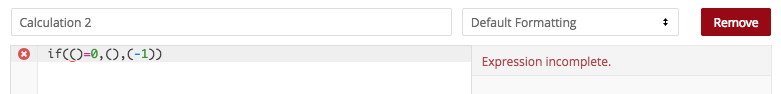...and we'll copy the first calculation, searching for the first slash...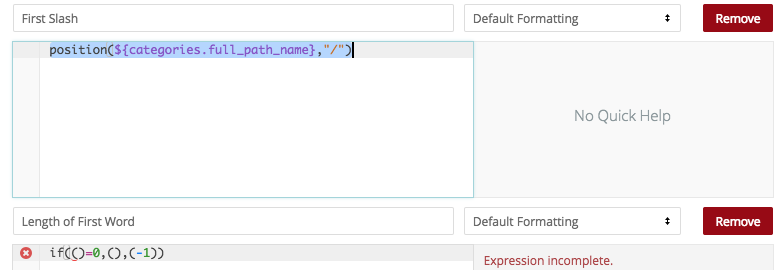...then paste it into the IF criteria below...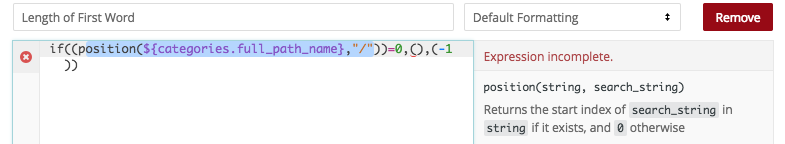now our positive results, if the position is zero, return the length of the full category...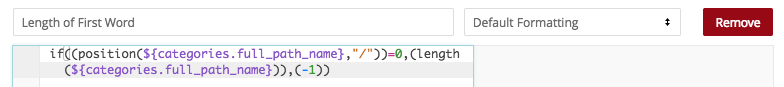then if the position is not zero, return the actual position...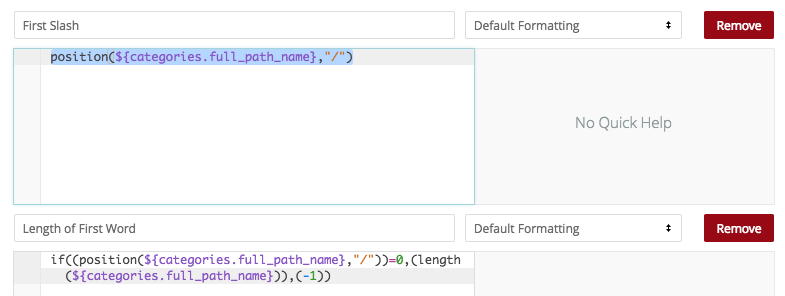...minus one...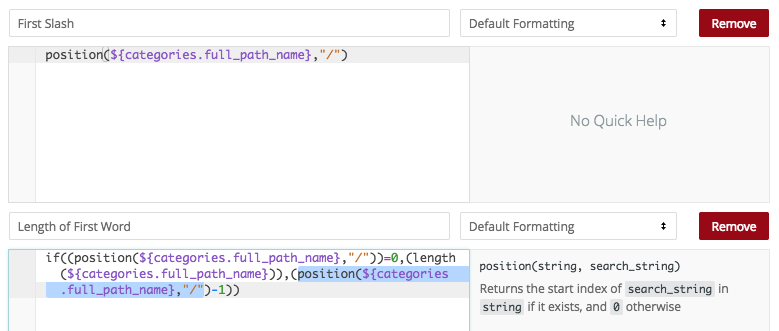So when we save, it shows us the length of the first word before any slashes...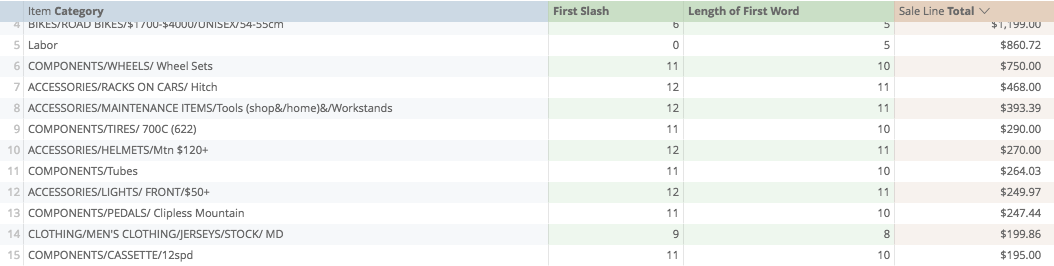Now we should test this, to determine that it is producing the desired effect. We can test it by starting the substring function...

Substring requires three arguments, what field is being looked at, where the substring begins, and where it ends...

What we're looking at and where it begins are easy: category, and position zero...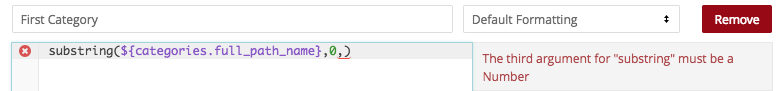Where it ends is what we're testing from the calculation above. Copying...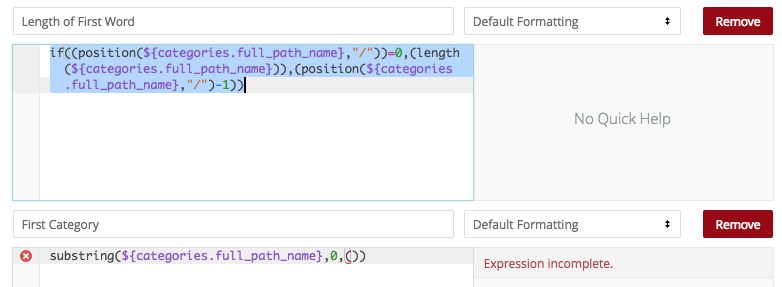...pasting...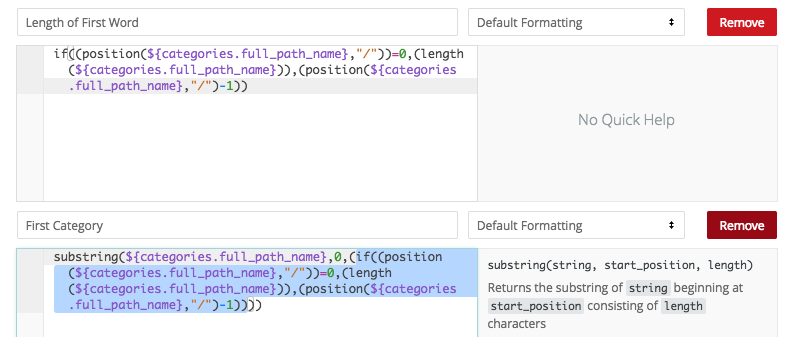...saving.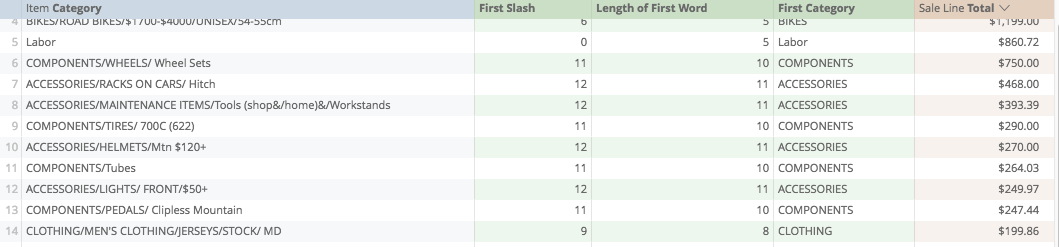We're checking for two things, are the words all complete? And are there any additional characters after the words (such as, the forward slash)

Our results look all right, so let's save our work so far...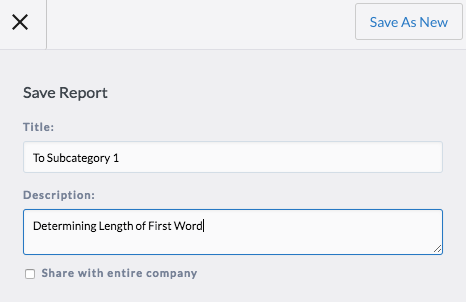## Step TwoWhat is the length of the forward slash?

This, we expect, should not be difficult to determine. Every slash would only be one character long if it is to be found at all.

We know that it's in a category if its position is greater than zero. We know it's not in a category if its position is zero.

So, this too, becomes an easy IF calculation...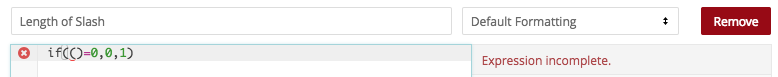And our source for its position comes from our first calculation...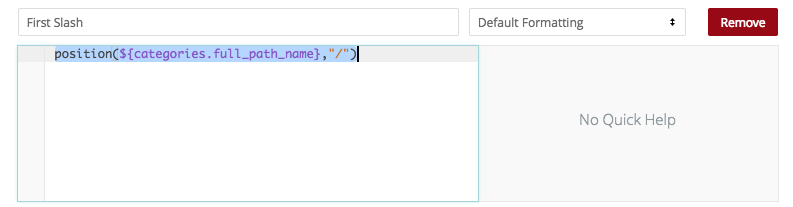Pasting...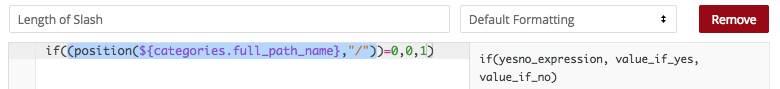And saving...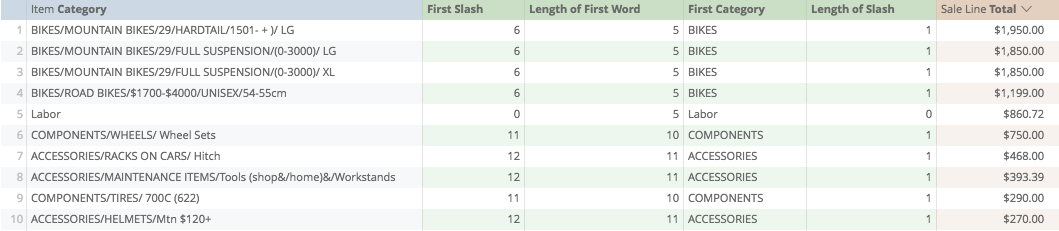Where it exists, we have a 1, where it doesn't we have a zero.

So far, so good. Let's save our work to here.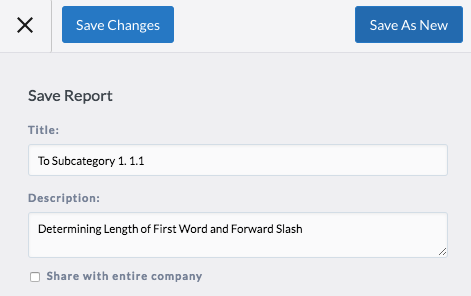## Step ThreeWhat is the length of the second word?

Now is where it gets a bit trickier.

Similarly to finding the length of the first word, we want to find the the place in the category where the second forward slash occurs. To do this, we want to rebuild the content after the first slash.

Again, we'll want to use the substring function to return this content, but instead of beginning at position zero, now we are starting at the position of where our first forward slash is.

BUT, if we start in that position, it will begin with the forward slash and then the subcategory. Eg: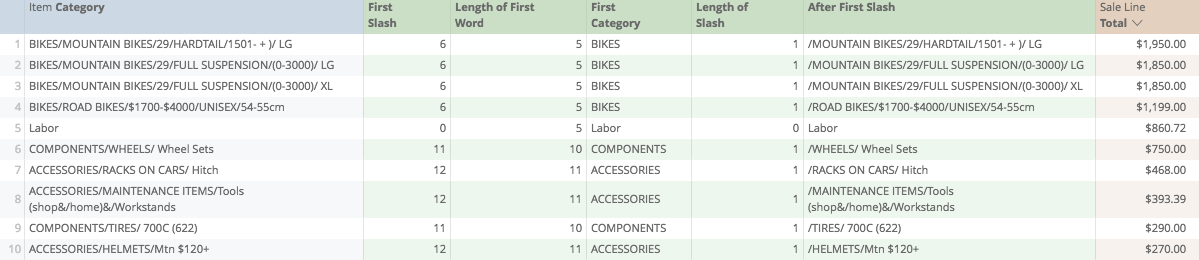What we want to do is start in the position plus one.

So, Substring...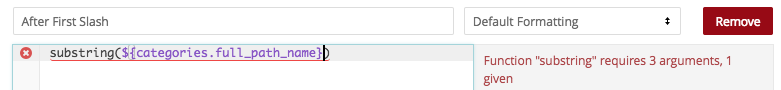...beginning...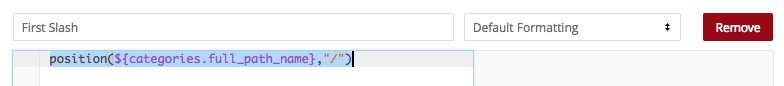...from our first position plus one...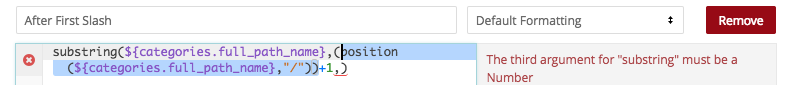...and then ending with just a really high number for a category length. I'm going to say 100, but this is arbitrary.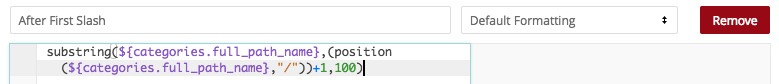So that when we save...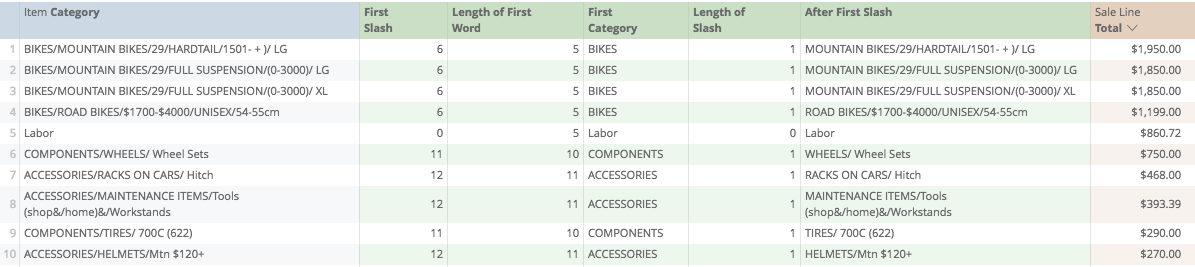We have all the content after the first forward slash.

(You may notice that "Labor" is still being returned as the content here. This is less of a problem than it looks. If we overshoot a complete category, it doesn't return an error. All we need to do is find if any further forward slashes occur, and trim the category there)

Let's save our work as new.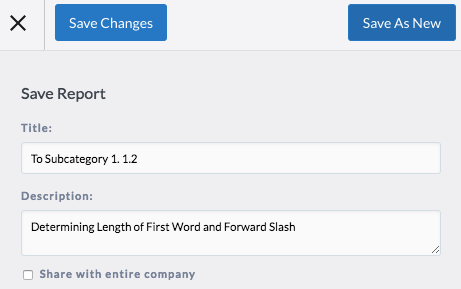Now, to complete this step, all we need to do is apply the same process as we did in step one. Beginning with the position of the slash...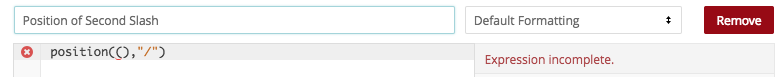in the remaining content...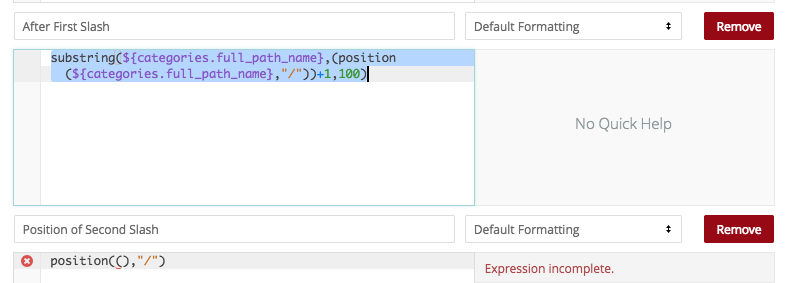so our final calculation looks like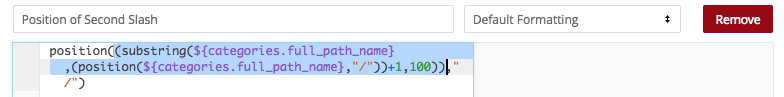And when we save, we get the same logic of numbers, 0 or some integer...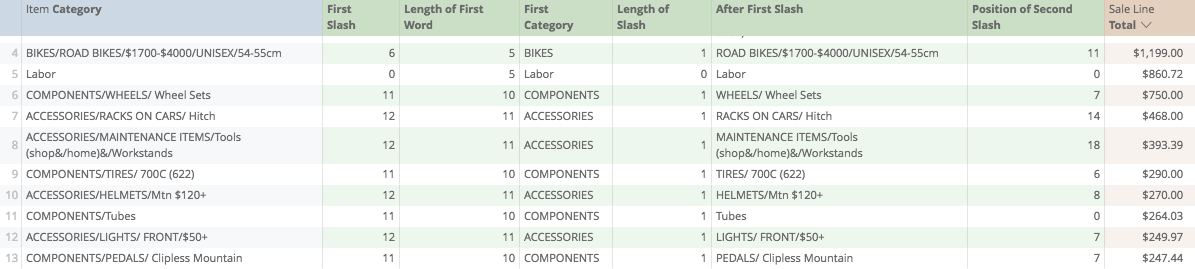Let's save as new.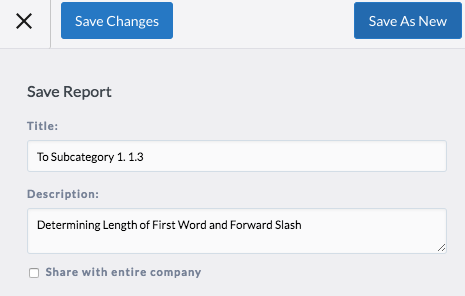And again, our if statement logic will be:

If the position of the second slash is zero, return the length of the remaining content.

If the position of the second slash is not zero, return the position of the slash minus one.

Starting: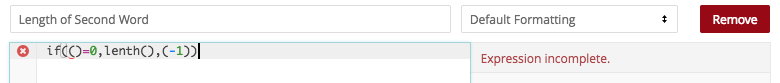If the position...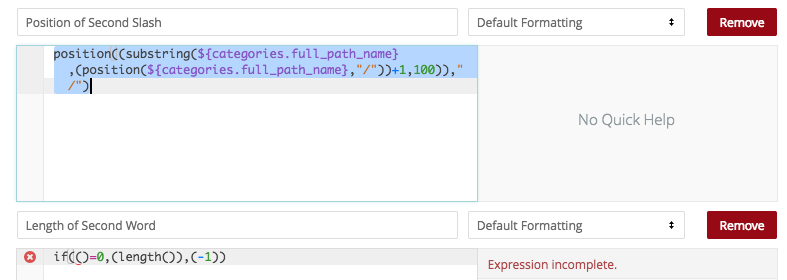is zero, return the length of the remaining content...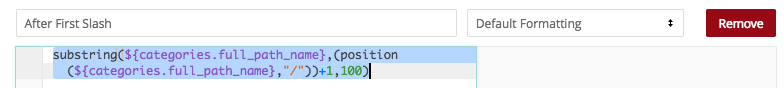...into the calculation...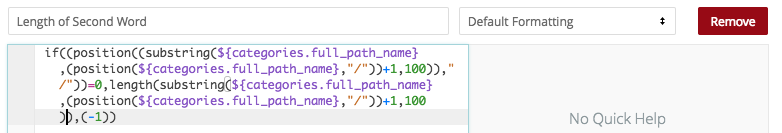if not, return the position of the slash...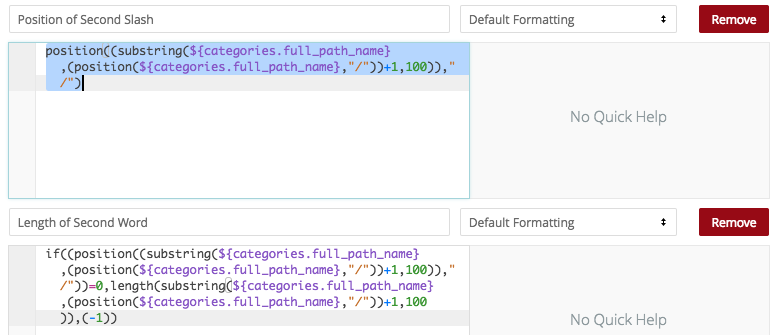minus one...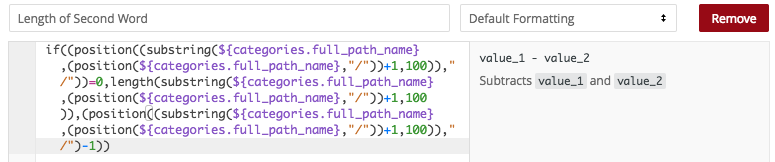So when we save...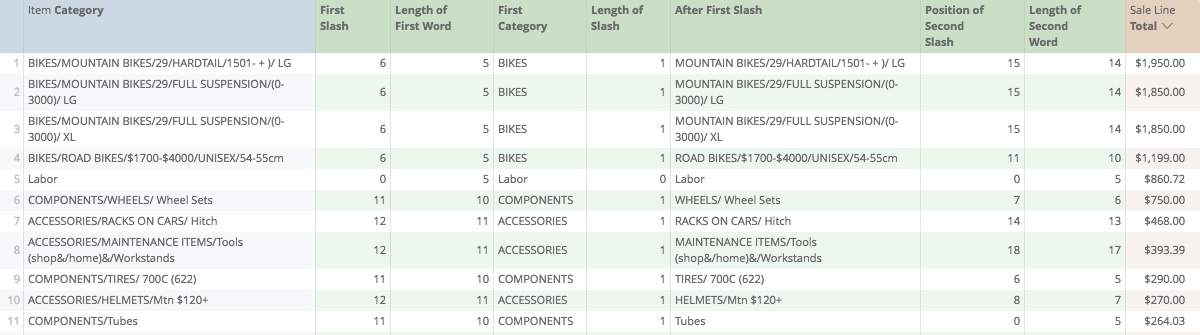We get the length of the second word.

Again we can test this...

Using Substring, we can look at the remaining content, starting at zero, and looking as far as the length of the second word.

For the sake of brevity, if you are curious, this is the calculation...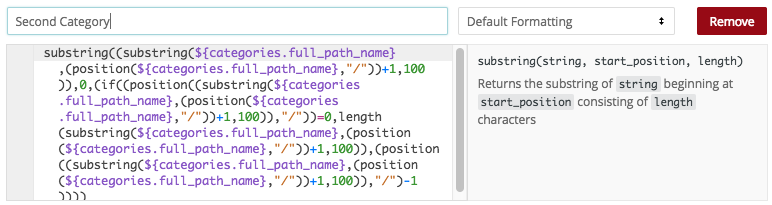and here it is in action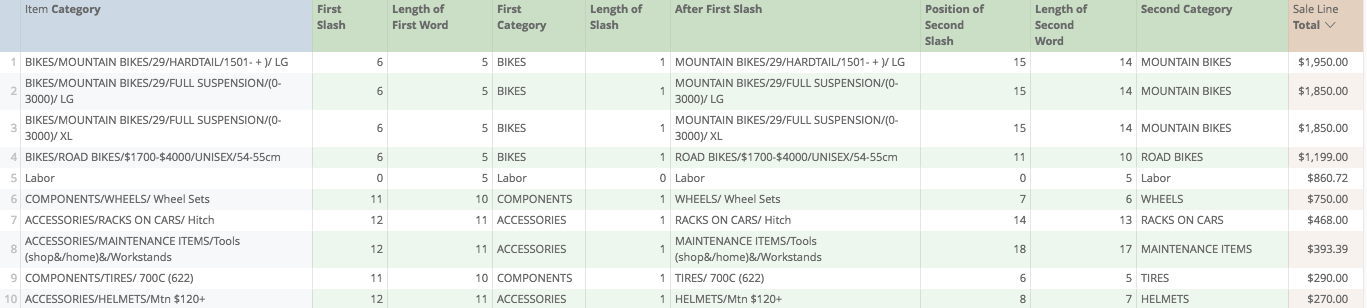So now we have determined the length of the first word, the length of the bracket, and the length of the final word.

## Step FourWhat is the length of all three?

Now a bit of a warning, our report is starting to get heavy with calculations. We should choose how we want to proceed from here. In this discussion, we are just trimming the categories to the first subcategory. so the only numbers we need now are Length of First Word, Length of Slash, and Length of Second Word.

Perhaps we would be interested in only retuning the category labels themselves, First Category and Second Category, for further analysis.

For the purposes of this discussion though, I'm going to remove all the calculations except the Length ones...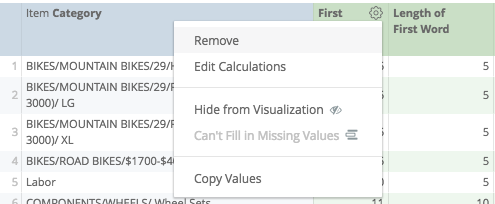...so our report should now look like...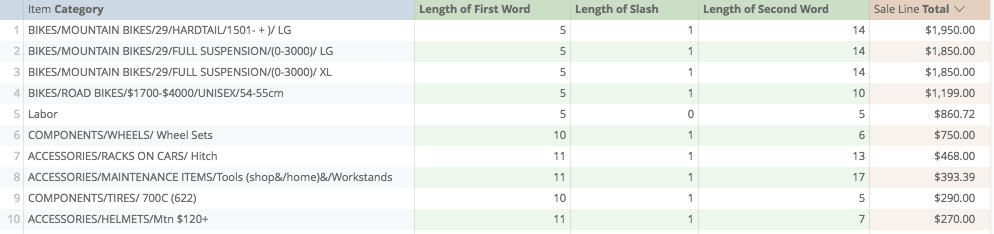and let's save as new...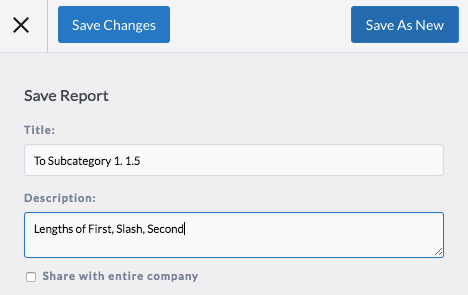Now, all we need to do is add the three calculations...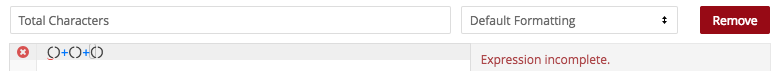First...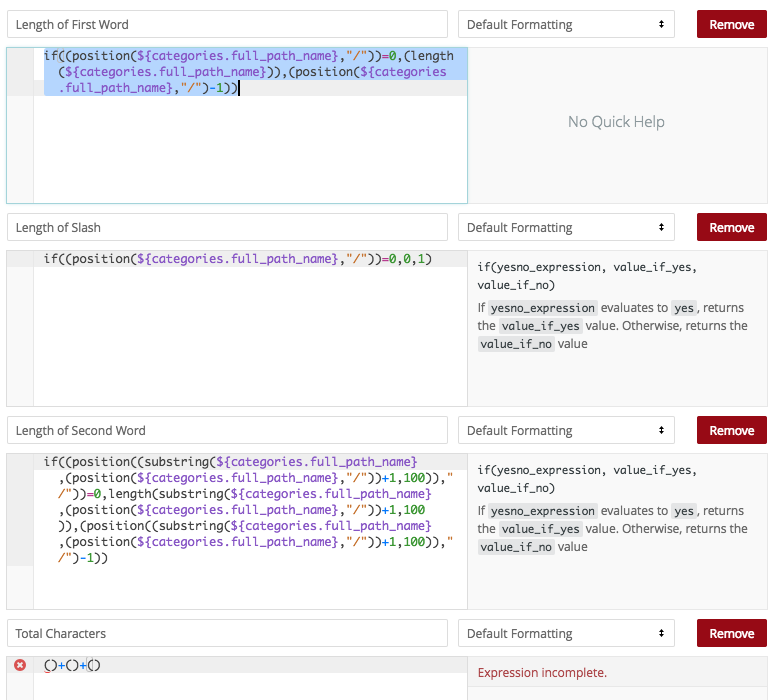plus...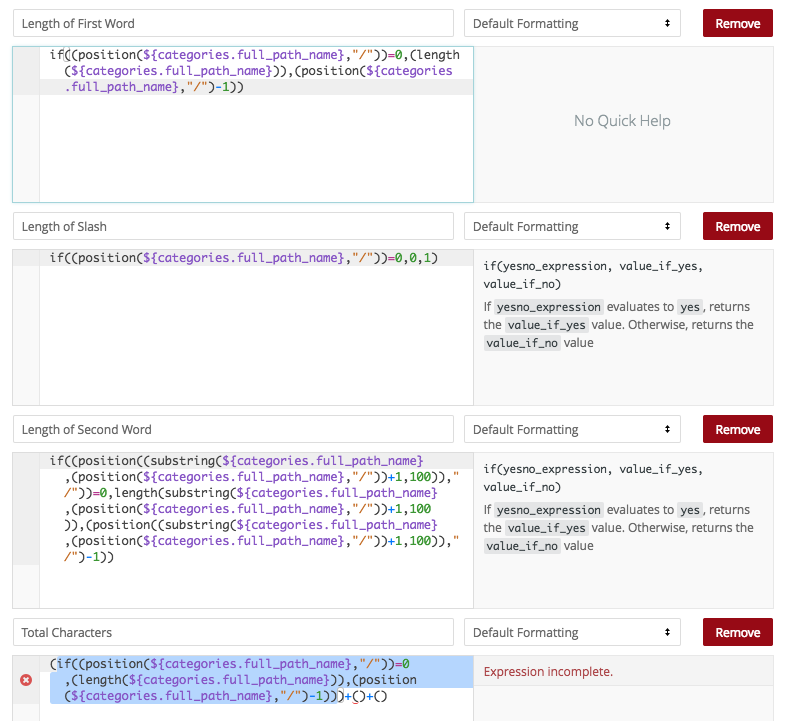second...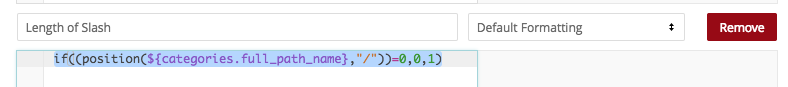plus...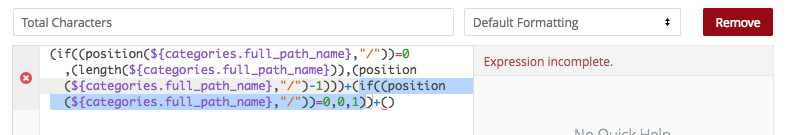third...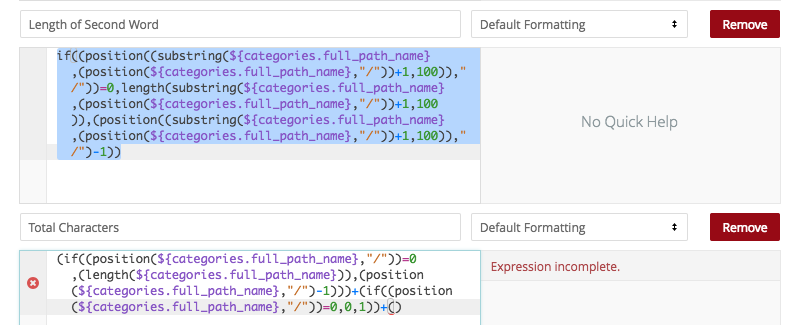and...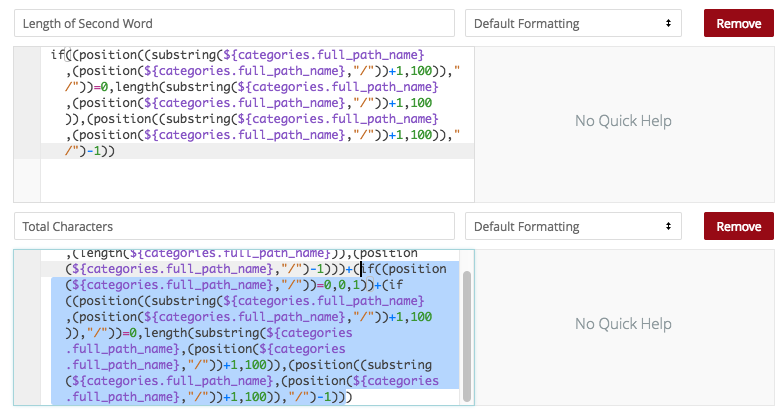when saving...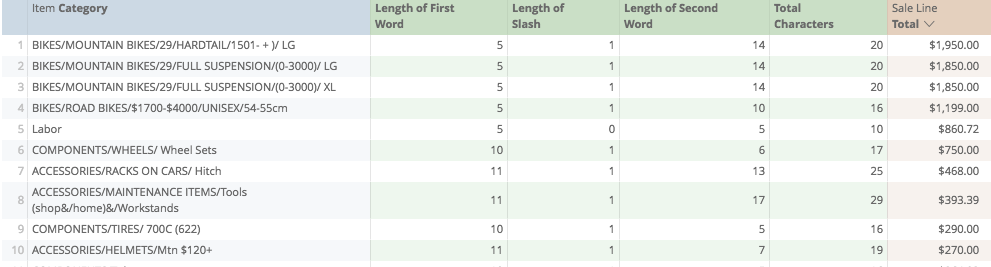We get one number.

Let's save this again...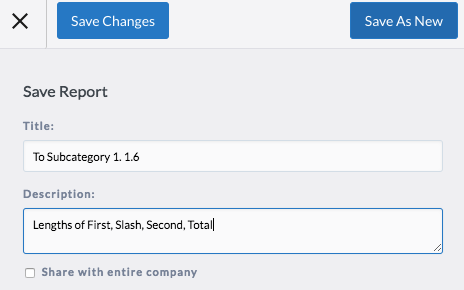And now, the Moment Of Truth.

Let's add a new calculation for substring of category...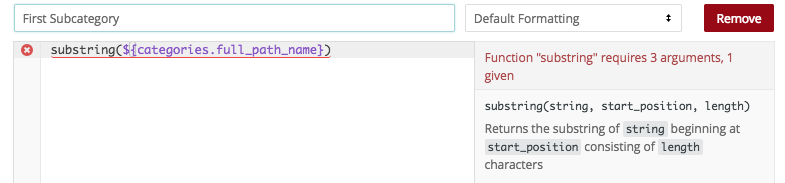starting in position zero...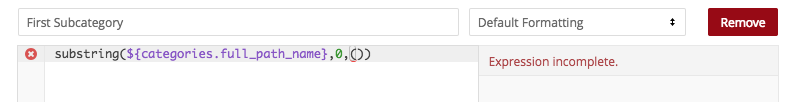then ending in our mess of calculation from the previous (too big to read now**) field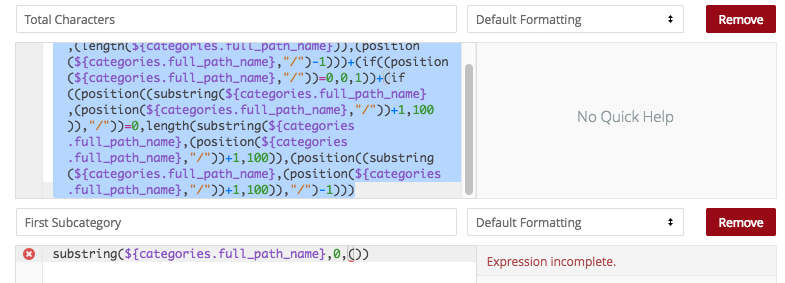pasting...and saving...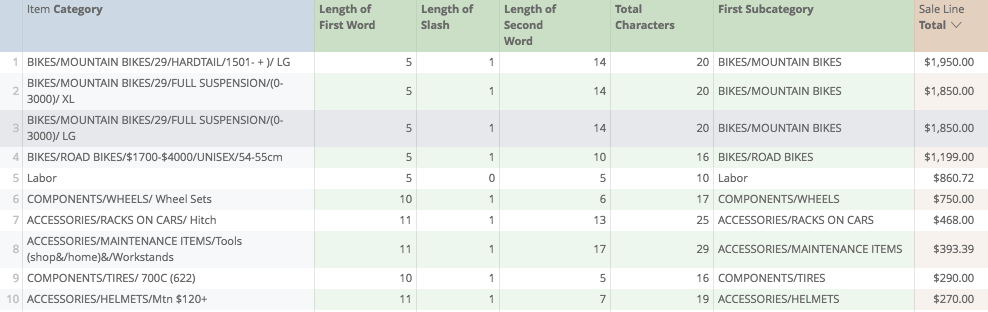and saving...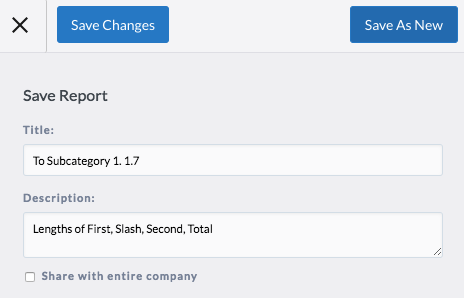So the work is done now!

Now, all we need to do is cosmetic. Let's remove all the calculations, except the last...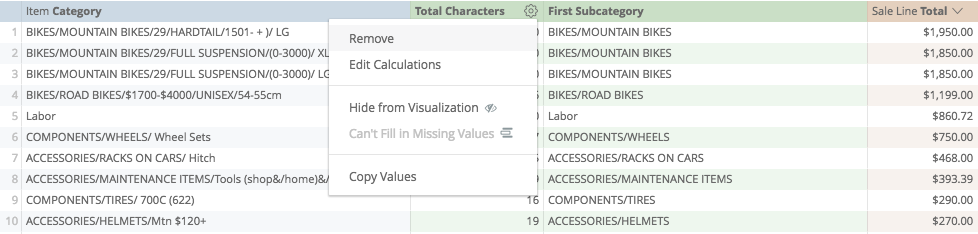And let's hide the Dimension of Category from Visualization...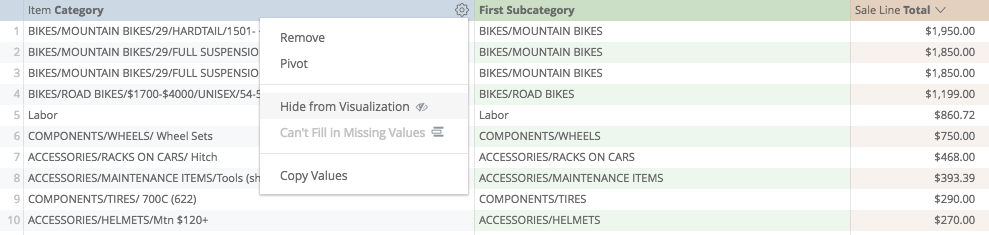Giving us a final data set that we can run, export, and then easily manipulate for further analysis!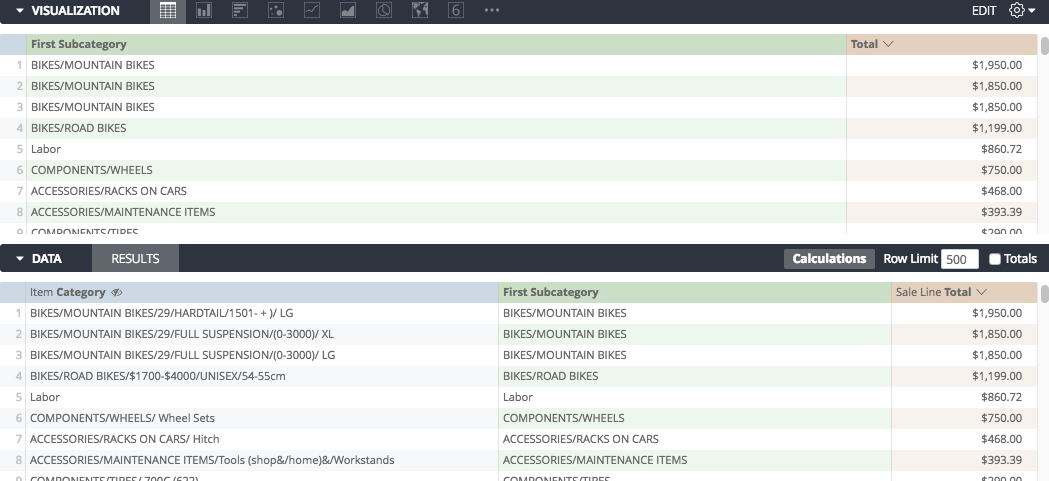*although I invite and welcome any improvements to be suggested to this process

**Spoiler Alert: Our final calculation here is:
substring(\${categories.full_path_name},0,((if((position(\${categories.full_path_name},"/"))=0,(length(\${categories.full_path_name})),(position(\${categories.full_path_name},"/")-1)))+(if((position(\${categories.full_path_name},"/"))=0,0,1))+(if((position((substring(\${categories.full_path_name},(position(\${categories.full_path_name},"/"))+1,100)),"/"))=0,length(substring(\${categories.full_path_name},(position(\${categories.full_path_name},"/"))+1,100)),(position((substring(\${categories.full_path_name},(position(\${categories.full_path_name},"/"))+1,100)),"/")-1)))))

Calculations Home

Joseph McKeown
Lightspeed Analytics and reporting consultant
Lightspeed HQ

## 1 comment

• edited October 2021

Can you give me the calculation to find JUST the 3rd level category as you did with the first and second? Because the visualization does not GROUP by the custom calculations and shows two rows for the same thing this report is still not very helpful on its own.

See above, I do not want "Helmet and Accessories" to appear twice, but rather once. Because I cannot do this with analytics I will need to export this file into excel to utilize their pivot tables to do this for me.

What I need to make this happen is Just the calculation for "Third Category" which I am missing in the image above. I do not need the first and second category grouped, but just the first, second, and third categories each on their own. Once I have this I can simply export to excel and do the rest on my own. Please provide me with the proper calculation to make that happen. Thank you.

edit:

I found that I can just take the same formula I used for "First Category" and "Second Category" and instead make them custom dimensions so that I avoid showing multiple lines for the same thing in visualization. However, I do still need a formula for the 3rd level category.

Post edited by SethM on